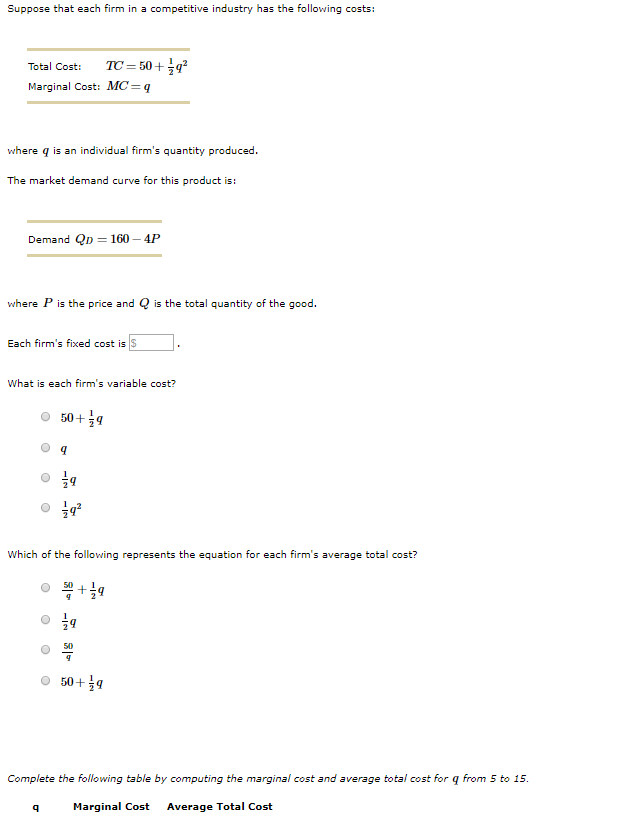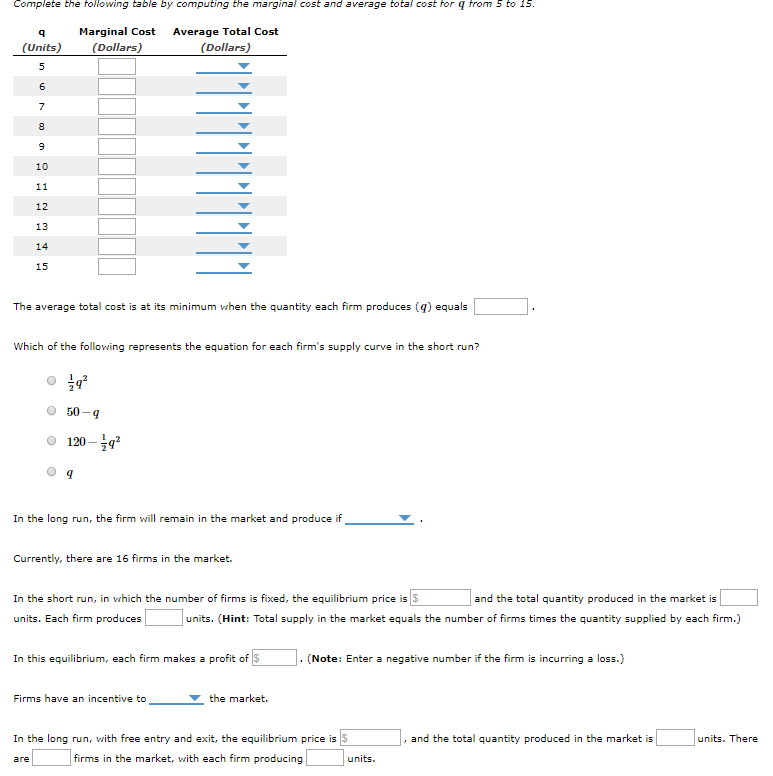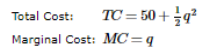# Suppose that each firm in a competitive industry has the following costs:TC = 50+ q?Total Cost:Marginal Cost: MC=qwhere q is an individual firm's quantity produced.The market demand curve for this product is:Demand Qp = 160 – 4Pwhere P is the price and Q is the total quantity of the good.Each firm's fixed cost isWhat is each firm's variable cost?50+9Which of the following represents the equation for each firm's average total cost?50+9Complete the following table by computing the marginal cost and average total cost for q from 5 to 15.Marginal CostAverage Total Cost Complete the following table by computing the marginal cost and average total cost for q from 5 to 15.Marginal Cost(Dollars)Average Total Cost(Units)(Dollars)101112131415The average total cost is at its minimum when the quantity each firm produces (g) equalsWhich of the following represents the equation for each firm's supply curve in the short run?50-4120 – 9In the long run, the firm will remain in the market and produce ifCurrently, there are 16 firms in the market.In the short run, in which the number of firms is fixed, the equilibrium price is Sand the total quantity produced in the market isunits. Each firm producesunits. (Hint: Total supply in the market equals the number of firms times the quantity supplied by each firm.)In this equilibrium, each firm makes a profit of S. (Note: Enter a negative number if the firm is incurring a loss.)Firms have an incentive tothe market.In the long run, with free entry and exit, the equilibrium price is S, and the total quantity produced in the market isunits. Therefirms in the market, with each firm producingunits.are

Question
206 viewshelp_outlineImage TranscriptioncloseSuppose that each firm in a competitive industry has the following costs: TC = 50+ q? Total Cost: Marginal Cost: MC=q where q is an individual firm's quantity produced. The market demand curve for this product is: Demand Qp = 160 – 4P where P is the price and Q is the total quantity of the good. Each firm's fixed cost is What is each firm's variable cost? 50+9 Which of the following represents the equation for each firm's average total cost? 50+9 Complete the following table by computing the marginal cost and average total cost for q from 5 to 15. Marginal Cost Average Total Cost fullscreenhelp_outlineImage TranscriptioncloseComplete the following table by computing the marginal cost and average total cost for q from 5 to 15. Marginal Cost (Dollars) Average Total Cost (Units) (Dollars) 10 11 12 13 14 15 The average total cost is at its minimum when the quantity each firm produces (g) equals Which of the following represents the equation for each firm's supply curve in the short run? 50-4 120 – 9 In the long run, the firm will remain in the market and produce if Currently, there are 16 firms in the market. In the short run, in which the number of firms is fixed, the equilibrium price is S and the total quantity produced in the market is units. Each firm produces units. (Hint: Total supply in the market equals the number of firms times the quantity supplied by each firm.) In this equilibrium, each firm makes a profit of S . (Note: Enter a negative number if the firm is incurring a loss.) Firms have an incentive to the market. In the long run, with free entry and exit, the equilibrium price is S , and the total quantity produced in the market is units. There firms in the market, with each firm producing units. are fullscreen
check_circle

Step 1

Hi, thank you for the question. As per our Honor Code, we can attempt only the first three subparts of the question. If you want solutions for other parts, kindly upload the question again specifying the parts to be solved.

Step 2

The following information is given:It is known that:

TC = TFC + TVC

TFC is the fixed cost that is not dependent on the output produced.

Hence, if the equation of TC is considered,

Firm’s fixed cost = \$50

TVC (variable cost) is the variable cost which varies as the output changes.

Hence, if the equation of TC is con...

### Want to see the full answer?

See Solution

#### Want to see this answer and more?

Solutions are written by subject experts who are available 24/7. Questions are typically answered within 1 hour.*

See Solution
*Response times may vary by subject and question.
Tagged in